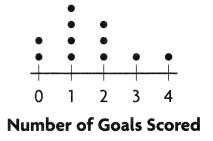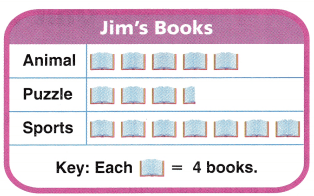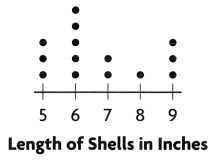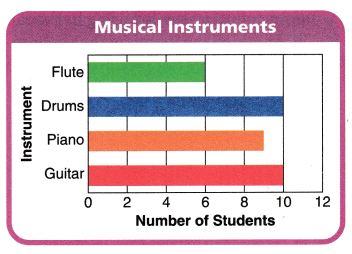Refer to our Texas Go Math Grade 3 Answer Key Pdf to score good marks in the exams. Test yourself by practicing the problems from Texas Go Math Grade 3 Unit 5 Assessment Answer Key.

Vocabulary

Choose the best term from the box.

Vocabulary
bar graph
dot plot
frequency table
pictograph

Question 1.
A ____________ uses dots to record each piece of data above a number line. (p. 647)
Explanation:
A Dot Plot is a graph for displaying the distribution of quantitative variable where each dot represents a value.

Question 2.
A ______________ is a table that uses numbers to record data. (p. 623)
Explanation:
Frequency refers to the number of times an event or a value occurs. A frequency table is a table that lists items and shows the number of times the items occur.

Question 3.
A __________ uses bars to show data. (p. 635)
Explanation:
A bar graph is a graph that shows complete data with rectangular bars and the heights of bars are proportional to the values that they represent

Concepts and Skills

Use the Dolphins Max Saw table for 4-6. (TEKS 3.8.A. 3.8.B)Question 4.
Max recorded in a table the number of dolphins he saw. How many dolphins did he see?
Explanation:
he saw 45 dolphins in all, Friday 12, Saturday 15, Sunday 19
12 + 15 + 19 = 46

Question 5.
How many more dolphins did Max see on Sunday than on Friday?
Explanation:
7  more dolphins did Max see on Sunday than on Friday.

Question 6.
If you made a bar graph of the data in the table, what labels would you use?
Answer: Rectangles to represent the data
Explanation:
If we made a bar graph of the data in the table the labels are Sunday, Saturday and Friday

Use the Number of Goals Scored dot plot for 7-8. (TEKS 3.8.A, 3.8.8)Question 7.
Katie recorded the number of goals the players on her team scored during soccer practice. How many players scored 2 goals?
Explanation:
Katie recorded the number of goals the players on her team scored during soccer practice.
3 players scored 2 goals

Question 8.
What was the least number of goals scored?
Explanation:
Zero was the score for 2 players

Fill in the bubble for the correct answer choice. Use the Jim’s Books pictograph for 9-13. (TEKS 3.8.A, 3.8.B)Question 9.
Jim made a pictograph to show the types of books he has. How many puzzle books does Jim have?
(A) 16
(B) 4
(C) 14
(D) 3
Explanation:
Jim have 14 puzzle books
it shows 3 and half
1 book whole = 4
4 x 3 = 12 and a half is 2
12 + 2 = 14

Question 10.
How many animal books does Jim have?
(A) 5
(B) 28
(C) 20
(D) 16
Explanation:
Jim have 20 animal books
5 x 4 = 20

Question 11.
How many books in all does Jim have?
(A) 15
(B) 62
(C) 20
(D) 31
Explanation:
Puzzle= 5 x 4 = 20
animal = 3 and half = 14
sports = 7 x 4 = 28
total = 62

Question 12.
How many more sports books than puzzle books does Jim have?
(A) 14
(B) 24
(C) 4
(D) 8
Explanation:
Number of puzzle books are 20
and number of sports books are 28
28 – 20 = 8 books

Question 13.
Which statement is true about the books Jim has?
(A) All of the books are puzzle books.
(B) None of the books is an animal book.
(C) Some of the books are computer books.
(D) Most of the books are sports or animal books.
Explanation:
There are no computer books in the graph as Jim has

Fill in the bubble for the correct answer choice. Use the Length of Shells in Inches dot plot for 14-17. (TEKS 3.8.A, 3.8.B)Question 14.
Robin collected shells during her vacation. She recorded the length of the shells to the nearest inch in a dot plot. How many shells were 9 inches long?
(A) 3
(B) 2
(C) 4
(D) 5
Explanation:
With the help of dot graph
there are 3 shells which  are 9 inches long

Question 15.
How many shells were 6 inches long or longer?
(A) 5
(B) 6
(C) 11
(D) 8
Explanation:
With the help of dot graph
5 shells are 6 inch longer

Question 16.
How many more shells did Robin collect that were 5 inches long than 8 inches long?
(A) 2
(B) 3
(C) 1
(D) 4
Explanation:
With the help of dot graph
2 more shells did Robin collect that were 5 inches long than 8 inches long

Question 17.
How many shells were 7 inches long or shorter?
(A) 5
(B) 6
(C) 2
(D) 10
Explanation:
2 shells were 7 inches long

Fill in the bubble for the correct answer choice. Use the Musical Instruments bar graph for 18-22. (TEKS 3.8.A, 3.8.B)Question 18.
Three more students play piano than which other instrument?
(A) flute
(B) drums
(C) violin
(D) guitar
Explanation:
Three more students play piano than Flute.

Question 19.
The same number of students play which two instruments?
(A) guitar and drums
(B) piano and drums
(C) drums and flute
(D) Not here
Explanation:
The same number of students play guitar and drums

Question 20.
How many fewer students play flute and piano combined than play drums and guitar combined?
(A) 11
(B) 14
(C) 5
(D) 6

Question 21.
How many more students play piano and guitar combined than play drums?
(A) 19
(B) 29
(C) 10
(D) 9